# Sum on dice

We have two dice. What is the greater likelihood of fall a total sum 7 or 8 ? (write 7, 8 or 0 if the probabilities are the same)?

x =  7

### Step-by-step explanation: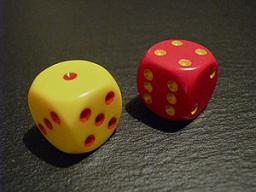Did you find an error or inaccuracy? Feel free to write us. Thank you!Math student
7=1+6=2+5=3+4=4+3=5+2=6+1
8=2+6=3+5=4+4=5+3=6+2
p1>p2
x=7Dr Math
hmmm, so we have 8 = 4+4 = 4+4 (thus one less variation than 7)Jay
i dont think soTips to related online calculators
Would you like to compute count of combinations?

## Related math problems and questions:

• Sum or productWhat is the probability that two dice fall will have the sum 7 or product 12?
• Fall sum or sameFind the probability that if you roll two dice, it will fall the sum of 10, or the same number will fall on both dice.
• Three diceThe player throwing the three dice asked G. Galilei the question: "Should I bet on the sum of 11 or the sum of 12?" What did Galilei answer him? Hint: write down all three triples of numbers that can be thrown and: have a total of 11 have a total of 12 an
• One dice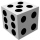Calculate the probability of one dice roll with the numbers 1, 2, 3, 4, 5, 6 on the walls. Write the results in a notebook in the shape of a fraction in the basic form: 2/3. a, The number 1 falls on the cube. b, The number 5 falls on the cube. c, An even
• Six on diceWhat is the probability that when throwing two dice will fall at least one six?
• Dices throwsWhat is the probability that the two throws of the dice: a) Six falls even once b) Six will fall at least once
• Dice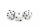How many times must throw the dice, the probability of throwing at least one six was greater than 90%?
• DiceWe throw five times the dice. What is the probability that six fits exactly twice?
• Sum of fall dices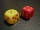What is the probability that the sum of 9 will fall on a roll of two dice? Hint: write down all the pairs that can occur as follows: 11 12 13 14 15. . 21 22 23 24. .. . 31 32. .. . . . . . .. . 66, count them, it's the variable n variable m: 36, 63,. .. .
• ThrowWe throw 2 times with 2 dices. What is the probability that the first roll will fall more than sum of 9 and the second throw have sum 3 or does not have the sum 4?
• Two diceWe roll two dice. What is the probability that the sum of the falling numbers is greater than 3?
• DiceWe throw 10 times a playing dice. What is the probability that the six will fall exactly 4 times?
• Sum 10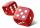What is the probability that two dice throw twice in a row will result the sum of 10?
• One threeWe throw two dice. What is the probability that max one three falls?
• The diceWhat is the probability of events that if we throw a dice is rolled less than 6?
• DicesWe will throw two dice. What is the probability that the ratio between numbers on first and second dice will be 1:2?
• Three reds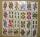What is the probability that when choosing 3 carats from seven carats, all 3 reds will be red?